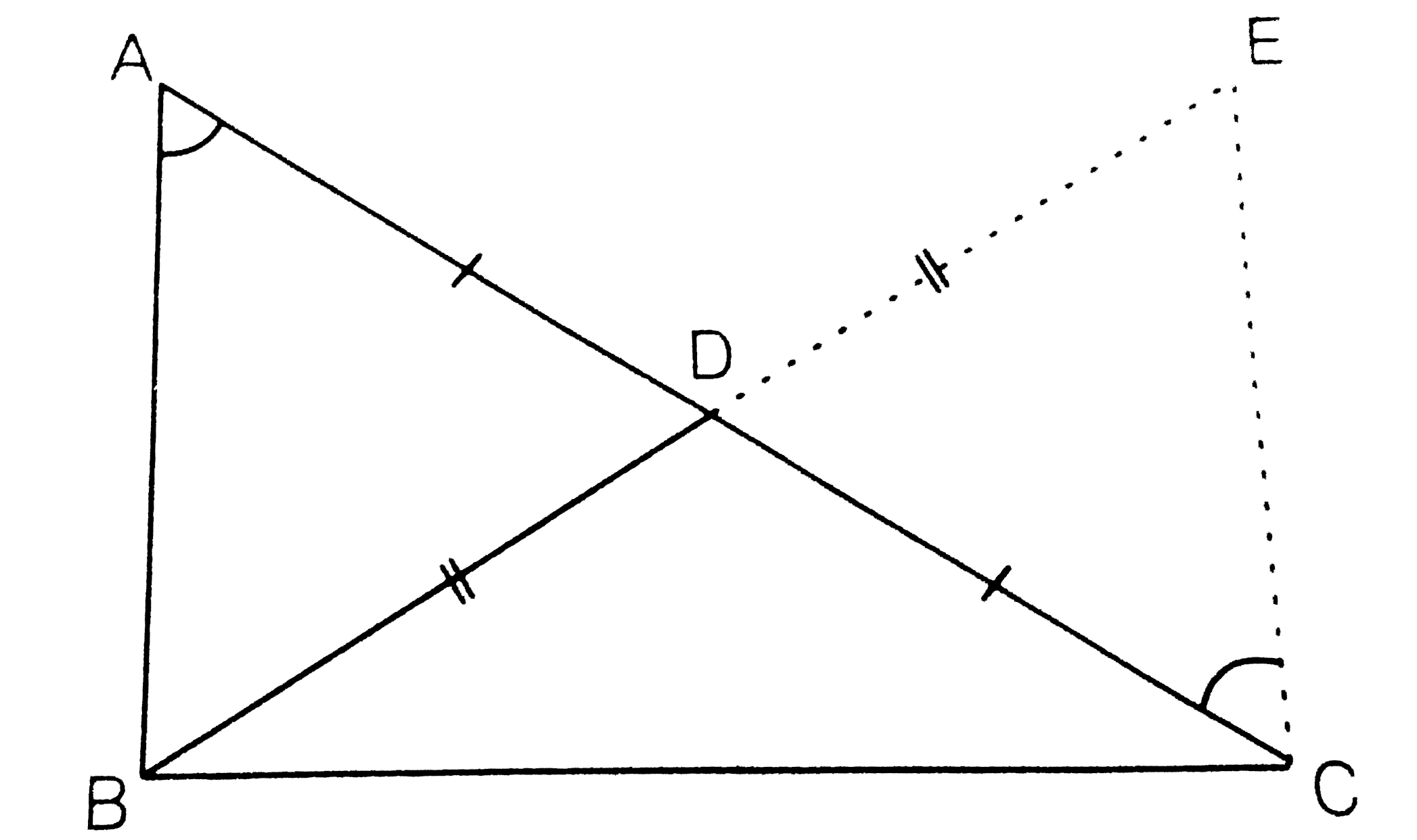Question

Updated on:26/04/2023

# In a right triangle,Prove that the line-segment joining the mid-point of the hypotenuse to the opposite vertex is half the hypotenuse

Text Solution

Solution
Given In ΔABC,B=90 and D is the mid-point of AC
Construction produce BD to E such that BD =DE and join EC
To ProveBD =DE [by construction ]
and ADB=CDE [vertically opposite angles ]
AB=EC [by CPCT]